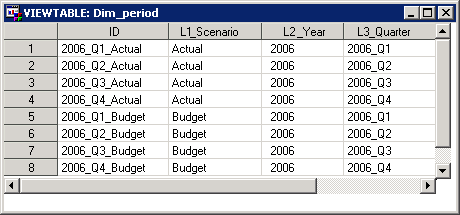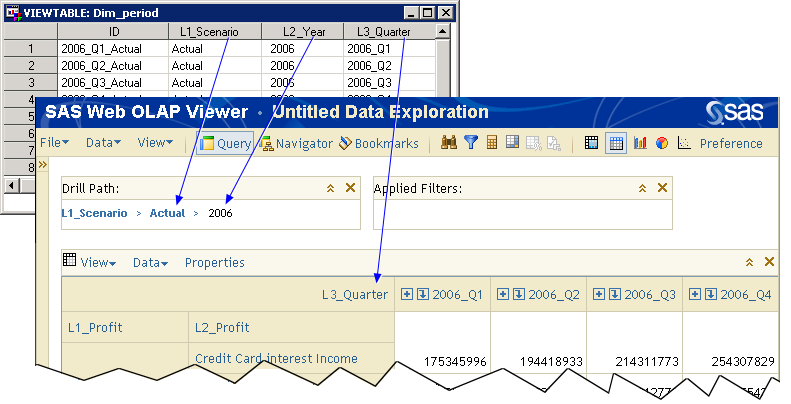# Period Dimensions Table

A period dimensions table defines the time periods to be used. The number of levels in the period dimensions table depends on your reporting needs. A period dimensions table contains the following columns and must conform to the following rules:

• The order of columns is significant.
• Each column must have the field length shown.
• The name of the column is arbitrary except for the ID column.
• The number of levels is arbitrary.
 Position Name Maximum Field Length Description 1 ID Char 32 The identifying value for the period Note: The name must be ID. 2 L1_Scenario Char 32 Top-level dimension member value (for example, Actual or Budget) 3 L2_Year Char 32 Second-level dimension member value (for example, 2008) 4 L3_Quarter Char 32 Third-level dimension member value (for example, 2008_Q4)

The following picture shows a sample period dimensions table:The following picture shows the relationship between this sample period dimensions table and the resulting profit-and-loss report: